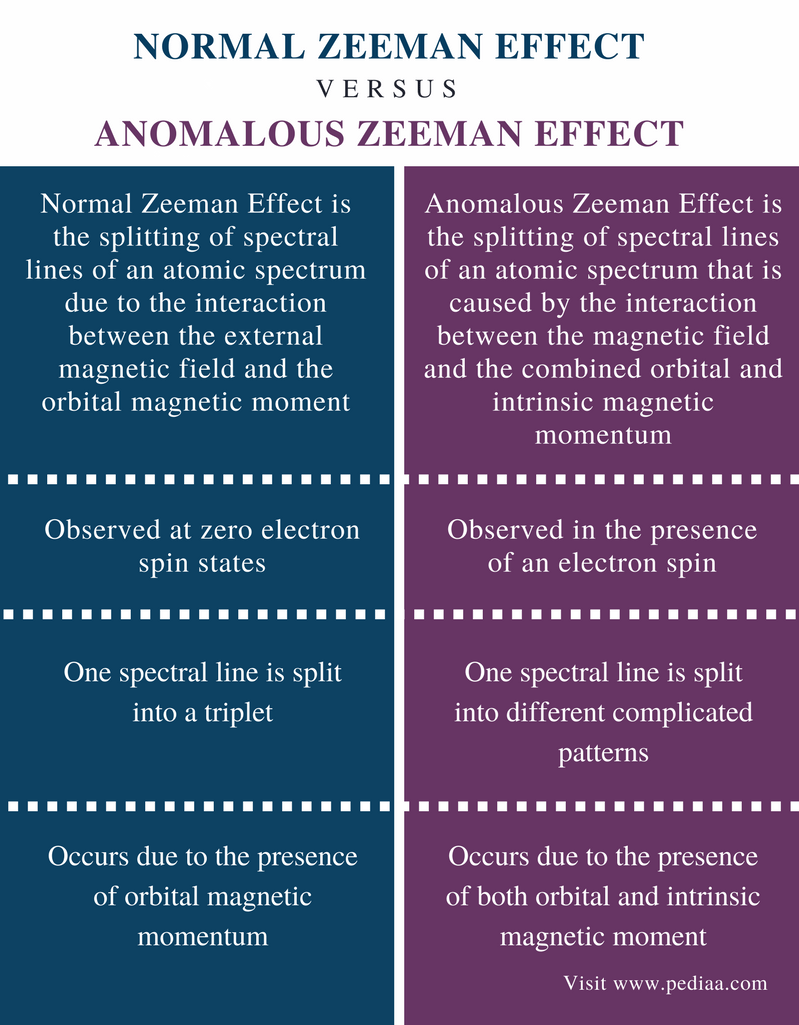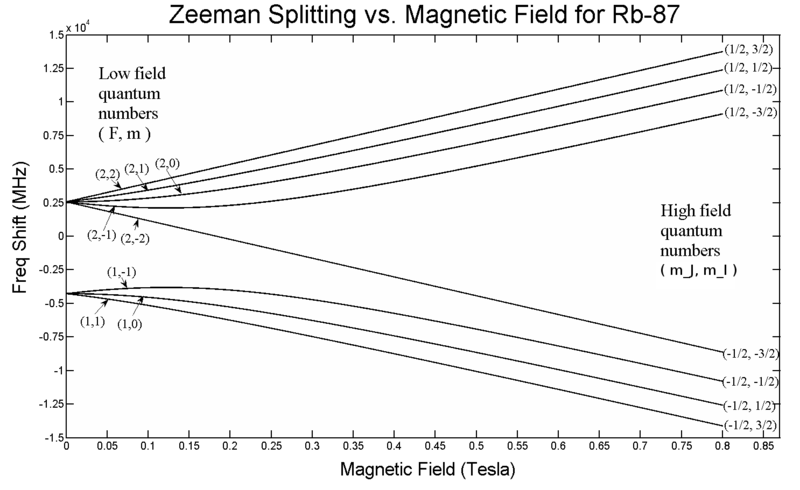# Difference Between Normal and Anomalous Zeeman Effect

## Main Difference – Normal vs Anomalous Zeeman Effect

Zeeman Effect is the splitting of spectral lines of an atomic spectrum in the presence of an external magnetic field. It is the result of the interaction between the magnetic momentum of the atom and the external magnetic field. Zeeman Effect can be observed in three types as normal Zeeman effect, anomalous Zeeman effect and diamagnetic Zeeman effect. The main difference between normal and the anomalous Zeeman effect is that normal Zeeman effect results in the formation of triplets by splitting a spectral line into three lines whereas anomalous Zeeman effect results in different splitting patterns from splitting of spectral lines.

### Key Areas Covered

1. What is Normal Zeeman Effect

– Definition, Explanation
2. What is Anomalous Zeeman Effect

– Definition, Explanation
3. What is the Difference Between Normal and Anomalous Zeeman Effect

– Comparison of Key Differences

Key Terms: Absorption Spectrum, Anomalous Zeeman Effect, Magnetic Field, Magnetic Momentum, Normal Zeeman Effect, Zeeman Effect## What is Normal Zeeman Effect

Normal Zeeman Effect is the splitting of spectral lines of an atomic spectrum due to the interaction between the external magnetic field and the orbital magnetic momentum. It is one of three types of Zeeman effect. This effect can be observed in the absence of electron spins.

When energy is given to an atom, the atom gains an excited state. The electrons of that atom can absorb energy and move to a higher energy level. Likewise, all electrons of that atom can absorb energy and move to higher energy levels. This gives us the absorption spectrum of that atom. Each spectral line indicates the energy difference between the energy levels that the electron moved through. The spectrum given in the normal condition is different from the spectrum given when the atom is placed in a magnetic field. It shows more spectral lines due to splitting.

The normal Zeeman effect can be observed for zero spin states. In zero spin state, the electron spin does not contribute to angular momentum. The normal Zeeman effect can be observed as a triplet in the observed spectrum instead of a single spectral line in the expected spectrum. There the single spectral line has been split into three lines with equal spaces between them.

## What is Anomalous Zeeman Effect

Anomalous Zeeman Effect is the splitting of spectral lines of an atomic spectrum caused by the interaction between magnetic field, the combined orbital and intrinsic magnetic moment. This effect can be observed as a complex splitting of spectral lines.

In some atoms, there are complex splitting patterns rather than triplet formations. This is the anomalous Zeeman effect. Here, the spectral lines are split into four lines, six lines, etc. Sometimes the spaces between the spectral lines are wider than expected. This happens due to the effects of electron spin. Since the spin of electrons contributes to the angular momentum, splitting becomes more complicated.Figure 1: Zeeman effect at Different Strengths of Magnetic Field

Moreover, the applied magnetic field has an effect on the splitting pattern of spectral lines. In weak fields, the splitting is much more similar to the normal Zeeman effect. But with the increased magnetic field, the splitting patterns also vary.

## Difference Between Normal and Anomalous Zeeman Effect

### Definition

Normal Zeeman Effect: Normal Zeeman Effect is the splitting of spectral lines of an atomic spectrum due to the interaction between the external magnetic field and the orbital magnetic moment.

Anomalous Zeeman Effect: Anomalous Zeeman Effect is the splitting of spectral lines of an atomic spectrum that is caused by the interaction between the magnetic field and the combined orbital and intrinsic magnetic momentum.

### Electron Spin

Normal Zeeman Effect: Normal Zeeman effect is observed at zero electron spin states.

Anomalous Zeeman Effect: The anomalous Zeeman effect is observed in the presence of an electron spin.

### Splitting Pattern

Normal Zeeman Effect: In normal Zeeman effect, one spectral line is split into a triplet.

Anomalous Zeeman Effect: In anomalous Zeeman effect, one spectral line is split into different complicated patterns.

### Magnetic Moment

Normal Zeeman Effect: Normal Zeeman effect occurs due to the presence of orbital magnetic momentum.

Anomalous Zeeman Effect: Anomalous Zeeman effect occurs due to the presence of both orbital and intrinsic magnetic moment.

### Conclusion

The phenomena of Zeeman effect describes the behavior of an atom in the presence of an external magnetic field. This Zeeman effect can be observed in two types as normal Zeeman effect and anomalous Zeeman effect. The main difference between normal and anomalous Zeeman effect is that normal Zeeman effect results in the formation of triplets by splitting a spectral line into three lines whereas anomalous Zeeman effect results in different splitting patterns from the splitting of spectral lines.

##### References:

1. “Zeeman Effect in Hydrogen.” Zeeman Effect, Available here.
2. “Zeeman effect.” Wikipedia, Wikimedia Foundation, 22 Oct. 2017, Available here.
3. PhysLink.com, Anton Skorucak. “What is the Zeeman effect?” PhysLink.com, Available here.

##### Image Courtesy:

1. “Breit-rabi-Zeeman” By Danski14 – Own work (CC BY-SA 3.0) via Commons Wikimedia